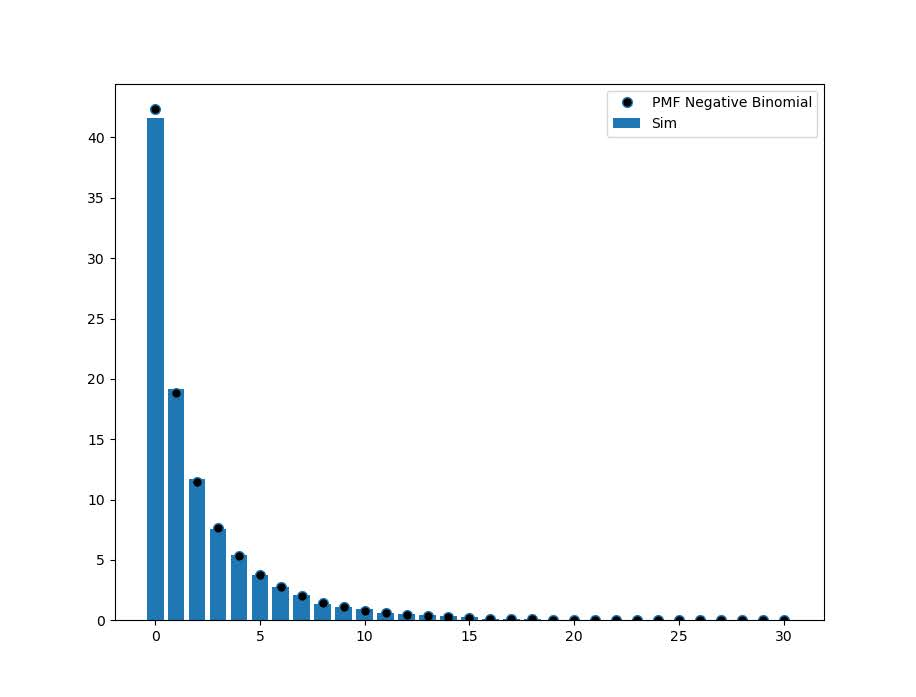# Simulating data with numpy and scipy

I’ve answered a few different questions on forums recently about simulating data:

I figured it would be worth my time to put some of my notes down in a blog post. It is not just academic, not too infrequently I use simulations at work to generate estimated return on investment estimates for putting a machine learning model into production. For social scientists this is pretty much equivalent to doing cost/benefit policy simulations.

Simulations are also useful for testing the behavior of different statistical tests, see prior examples on my blog for mixture trajectories or random runs.

Here I will be showing how to mostly use the python libraries numpy and scipy to do various simulation tasks. For some upfront (note I set the seed in numpy, important for reproducing your simulations):

``````import itertools as it
import numpy as np
import pandas as pd
np.random.seed(10)

# total simulations for different examples
n = 10000

# helper function to pretty print values
def pu(x,ax=1):
te1,te2 = np.unique(x.sum(axis=ax),return_counts=True)
te3 = 100*te2/te2.sum()
res = pd.DataFrame(zip(te1,te2,te3),columns=['Unique','Count','Percent'])
return res``````

## Sampling from discrete choice sets

First, in the linked data science post, the individual had a very idiosyncratic set of simulations. Simulate a binary vector of length 10, that had 2,3,4 or 5 ones in that vector, e.g. `[1,1,0,0,0,0,0,0,0,0]` is a valid solution, but `[0,0,0,0,1,0,0,0,0,0]` is not, since the latter only has 1 `1`. One way to approach problems like these is to realize that the valid outcomes are a finite number of discrete solutions. Here I use itertools to generate all of the possible permutations (which can easily fit into memory, only 627). Then I sample from that set of 627 possibilities:

``````# Lets create the whole sets of possible permutation lists
res = []
zr = np.zeros(10)
for i in range(2,6):
for p in it.combinations(range(10),i):
on = zr.copy()
on[list(p)] = 1
res.append(on.copy())

resnp = np.stack(res,axis=0)

# Now lets sample 1000 from this list
total_perms = resnp.shape
samp = np.random.choice(total_perms,n)
res_samp = resnp[samp]

# Check to make sure this is OK
pu(res_samp)``````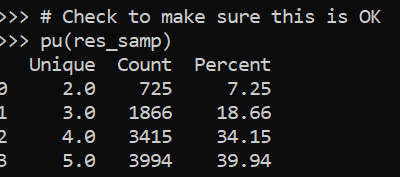Ultimately you want the simulation to represent reality. So pretend this scenario was we randomly pick a number out of the set {2,3,4,5}, and then randomly distribute the 1s in that length 10 vector. In that case, this sampling procedure does not reflect reality, because 2’s have fewer potential permutations than do 5’s. You can see this in the simulated proportions of rows with 2 (7.25%) vs rows with 5 (39.94%) in the above simulation.

We can fix that though by adjusting the sampling probabilities from the big set of 627 possibilities though. Pretty much all of the `np.random` methods an option to specify different marginal probabilities, where in choice it defaults to equal probability.

``````# If you want the different subsets to have equal proportions
sum_pop = resnp.sum(axis=1)
tot_pop = np.unique(sum_pop,return_counts=True)
equal_prop = 1/tot_pop.shape
pop_prob = pd.Series(equal_prop/tot_pop,index=tot_pop)
long_prob = pop_prob[sum_pop]

samp_equal = np.random.choice(total_perms,n,p=long_prob)
res_samp_equal = resnp[samp_equal]
pu(res_samp_equal)``````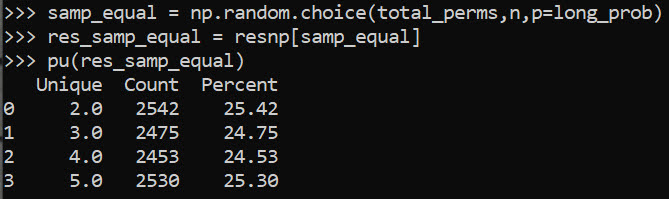So now we can see that each of those sets of results have similar marginal proportions in the simulation.

You can often figure out exact distributions for your simulations, for an example of similar discrete statistics, see my work on small sample Benford tests. But I often use simulations to check my math even if I do know how to figure out the exact distribution.

Another trick that I commonly use in other applications that don’t have access to something equivalent to `np.random.choice`, but most applications have a random uniform generator. Basically you can generate random numbers on whatever interval, chunk those up into bits, and turn those bits into your resulting categories. This is what I did in that SPSS post at the beginning.

``````unif = np.floor(np.random.uniform(1,33,(32*n,1)))/2
pu(unif)``````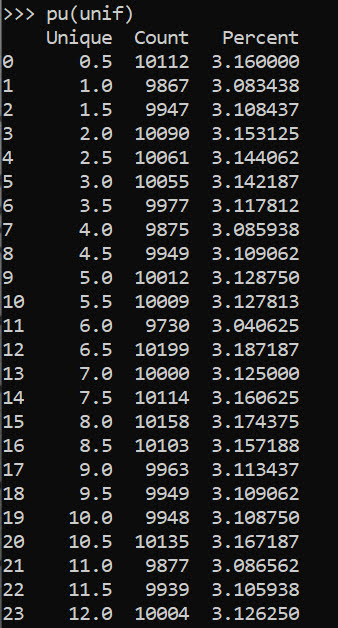But again this is not strictly necessary in python because we can generate the set/list of items and sample from that directly.

``````# Same as using random choice from that set of values
half_vals = np.arange(1,33)/2
unif2 = np.random.choice(half_vals,(32*n,1))
pu(unif2)``````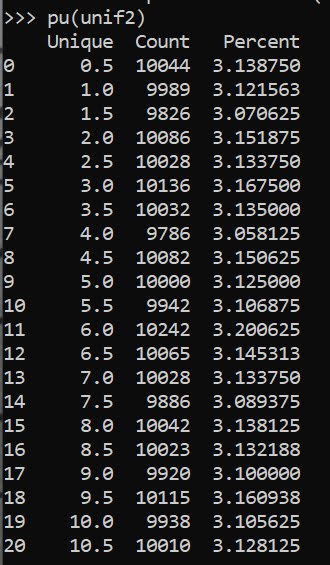But if limited in your libraries that is a good trick to know. Note that most random number generators operate over `(0,1)`, so open intervals and will never generate an exact 0 or 1. To get a continuous distribution over whatever range, you can just multiply the 0/1 random number generator (and subtract if you need negative values) to match the range you want. But again most programs let you input min/max in a uniform number generator.

## Sampling different stat distributions

So real data often can be reasonably approximated via continuous distributions. If you want to generate different continuous distribtions with approximate correlations, one approach is to:

• generate multi-variate standard normal data (mean 0, variance 1, and correlations between those variables)
• turn that matrix into a standard uniform via the CDF function
• then for each column, use the inverse CDF function for the distribution of choice

Here is an example generating a uniform 0/1 and a Poisson with a mean of 3 variable using this approach.

``````from scipy.stats import norm, poisson

# Here generate multivariate standard normal with correlation 0.2
# then transform both to uniform
# Then transform 2nd column to Poisson mean 3

mu = [0,0]
cv = [[1,0.2],[0.2,1]] #needs to be symmetric
mv = np.random.multivariate_normal([0,0],cov=cv,size=n)
umv = pd.DataFrame(norm.cdf(mv),columns=['Uniform','Poisson'])
umv['Poisson'] = poisson.ppf(umv['Poisson'],3)
print(umv.describe())``````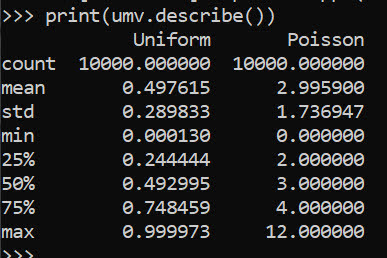This of course doesn’t guarantee the transformed data has the exact same correlation as the original specified multi-variate normal. (If interested in more complicated scenarios, it will probably make sense to check out copulas.)

Like I mentioned in the beginning, I am often dealing with processes of multiple continuous model predictions. E.g. one model that predicts a binary ‘this claim is bad’, and then a second model predicting ‘how bad is this claim in terms of \$\$\$’. So chaining together simulations of complicated data (which can be mixtures of different things) can be useful to see overall behavior of a hypothetical system.

Here I chain together a predicted probability for 3 claims (20%,50%,90%), and mean/standard deviations of (1000,100),(100,20),(50,3). So pretend we can choose 1 of these three claims to audit. We have the predicted probability that the claim is wrong, as well as an estimate of how much money we would make if the claim is wrong (how wrong is the dollar value).

The higher dollar values have higher variances, so do you pick the safe one, or do you pick the more risky audit with higher upside. We can do a simulation to see the overall distribution:

``````pred_probs = np.array([0.2,0.5,0.9])
bin_out = np.random.binomial(n=1,p=pred_probs,size=(n,3))
print( bin_out.mean(axis=0) )

# Pretend have both predicted prob
# and distribution of values
# can do a simulation of both

val_pred = np.array([1000,100,50])
val_std = np.array([100,20,3])
val_sim = np.random.normal(val_pred,val_std,size=(n,3))
print(val_sim.mean(axis=0))
print(val_sim.std(axis=0))

revenue = val_sim*bin_out
print(revenue.mean(axis=0))
print(revenue.std(axis=0))``````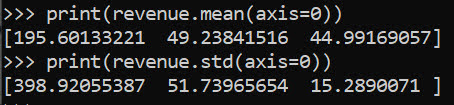I could see a reasonably risk averse person picking the lowest dollar claim here to audit. Like I said in the discrete case, we can often figure out exact distributions. Here the expected value is easy, `prob*val`, the standard deviation is alittle more tricky to calculate in your head (it is a mixture of a spike at 0 and then the rest of the typical distribution):

``````# Theoretical mean/variance
expected = pred_probs*val_pred
low_var = (expected**2)*(1-pred_probs)
hig_var = ((val_pred - expected)**2)*pred_probs
std_exp = np.sqrt(low_var + hig_var)``````

But it still may be useful to do the simulation here anyway, as the distribution is lumpy (so just looking at mean/variance may be misleading).

Other common continuous distributions I use are beta, to simulate between particular endpoints but not have them uniform. And negative binomial is a good fit to not only many count distributions, but things that are more hypothetically continuous (e.g. for distances instead of gamma, or for money instead of log-normal).

Here is an example generating a beta distribution between 0/1, with more of the distribution towards 0 than 1:

``````# mean probability 0.2
a,b = 1,5
beta_prob = beta.rvs(a, b, size=n)
plt.hist(beta_prob, bins=100,density=True,label='Sim')
# theoretical PDF
x = np.arange(0,1.01,0.01)
plt.plot(x,beta.pdf(x,a,b),label=f'beta({a},{b}) PDF')
plt.legend()
plt.show()``````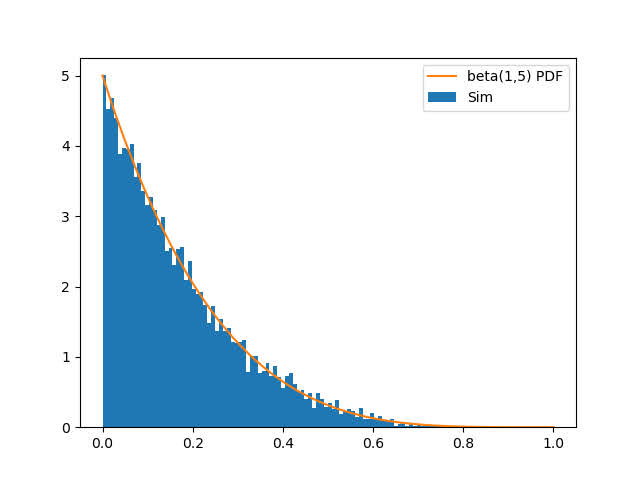For beta, the parameters a,b, the mean is a/b. To make the distribution more spread out, you have larger overall values of a/b (I don’t have the conversion to variance memorized offhand). But if you have real data, you can plop that into scipy to fit the parameters, here I fix the location and scale parameters.

``````# If you want to fit beta based on observed data
fitbeta = beta.fit(beta_prob,floc=0,fscale=1)``````

We can do similar for negative binomial, I often think of these in terms of regression dispersion parameters, and I have previously written about translating mean/dispersion to n/p notation:

``````# Ditto for negative binomial
mean_nb = 2
disp_nb = 4

def trans_np(mu,disp):
x = mu**2/(1 - mu + disp*mu)
p = x/(x + mu)
n = mu*p/(1-p)
return n,p

nb_n,nb_p = trans_np(mean_nb,disp_nb)
nb_sim = nbinom.rvs(nb_n,nb_p,size=(n,1))
nb_bars = pu(nb_sim)
plt.bar(nb_bars['Unique'],nb_bars['Percent'],label='Sim')

x = np.arange(0,nb_sim.max()+1)
plt.plot(x,nbinom.pmf(x,nb_n,nb_p)*100,linestyle='None',
marker='o',markerfacecolor='k',ms=7,
label=f'PMF Negative Binomial')
plt.legend()
plt.show()``````